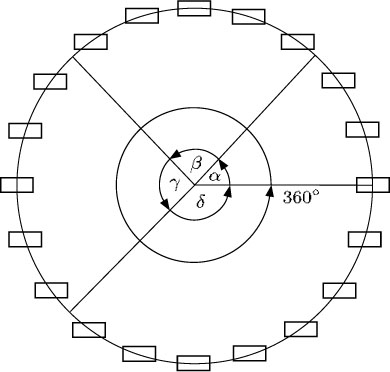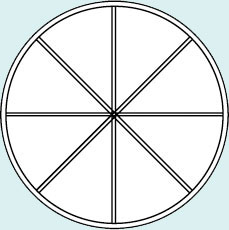Science, Maths & Technology

### Become an OU studentGeometry

Start this free course now. Just create an account and sign in. Enrol and complete the course for a free statement of participation or digital badge if available.

# 1.3.1 Angles at a point

Another useful property to remember is that one complete turn is 360°. This means that when there are several angles making up a complete turn, the sum of those angles must be 360°.

For instance, if the angles turned by a Big Wheel at a fairground as it picks up passengers were α, β, γ and δ as shown in the diagram below, then α + β + γ + δ = 360°.The sum of angles at a point is 360°.

## Example 1

Calculate the angle between adjacent spokes of this wheel.